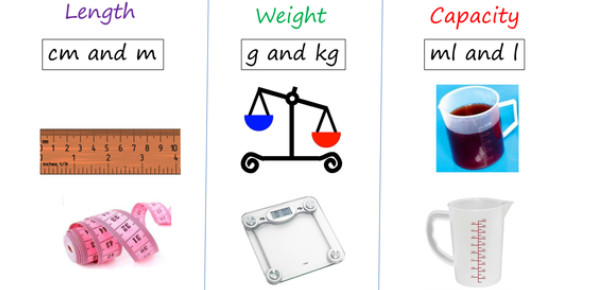# Math Quiz - Capacity, Weight And Length For 6th Grade

15 Questions | Attempts: 115688SettingsThe quiz below is designed for a sixth grade math class. It covers capacity, length, weight and temperature. Do you believe you can answer them all truthfully? Test out how well informed you are when it comes to these math problems by giving it a try. All the best as you do!

• 1.
1 cup (c) = _____ ounces (oz)
• A.

8 ounces

• B.

20 ounces

• C.

2 ounces

• D.

10 ounces

• E.

50 ounces

• 2.
____ cups (c) = 1 pint (pt)
• A.

1 cup

• B.

15 cups

• C.

2 cups

• D.

10 cups

• E.

16 cups

• 3.
____ cups(c)  = 1 quart (qt)
• A.

5 cups

• B.

8 cups

• C.

9 cups

• D.

1 cup

• E.

4 cups

• 4.
______ pints (pt) = 1 quart (qt)
• A.

16 pints

• B.

8 pints

• C.

2 pints

• D.

5 pints

• E.

4 pints

• 5.
____ quarts (qt) = 1 gallon (gal)
• A.

1 quart

• B.

8 quarts

• C.

12 quarts

• D.

4 quarts

• E.

15 quarts

• 6.
___ inches (in) = 1 foot (ft)
• A.

12 inches

• B.

8 inches

• C.

24 inches

• D.

36 inches

• E.

10 inches

• 7.
____ feet (ft) = 1 yard
• A.

6 feet

• B.

2 feet

• C.

12 feet

• D.

3 feet

• E.

70 feet

• 8.
1 yard (yd) = ______ inches (in)
• A.

24 inches

• B.

12 inches

• C.

48 inches

• D.

36 inches

• E.

70 inches

• 9.
1 mile (mi) = ______________ feet (ft)
• A.

6000 feet

• B.

2800 feet

• C.

3000 feet

• D.

7600 feet

• E.

5280 feet

• 10.
1 mile (mi) = ______________ yards
• A.

3625 yards

• B.

1760 yards

• C.

1258 yards

• D.

4259 yards

• E.

7825 yards

• 11.
1 pound (lb) = ________ounces (oz)
• A.

16 ounces

• B.

12 ounces

• C.

8 ounces

• D.

24 ounces

• E.

10 ounces

• 12.
1 ton = ________ pounds (lb)
• A.

7,625 pounds

• B.

3,258 pounds

• C.

4,000 pounds

• D.

2,000 pounds

• E.

1,500 pounds

• 13.
____ ounces(oz)  = 1 pint (pt)
• A.

8 ounces

• B.

12 ounces

• C.

36 ounces

• D.

10 ounces

• E.

16 ounces

• 14.
_______ ounces (oz) = 1 quart (qt)
• A.

48 ounces

• B.

24 ounces

• C.

32 ounces

• D.

40 ounces

• E.

55 ounces

• 15.
_____ pints = 1 gallon
• A.

20 pints

• B.

8 pints

• C.

5 pints

• D.

10 pints

• E.

16 pints

## Related TopicsBack to top
×

Wait!
Here's an interesting quiz for you.